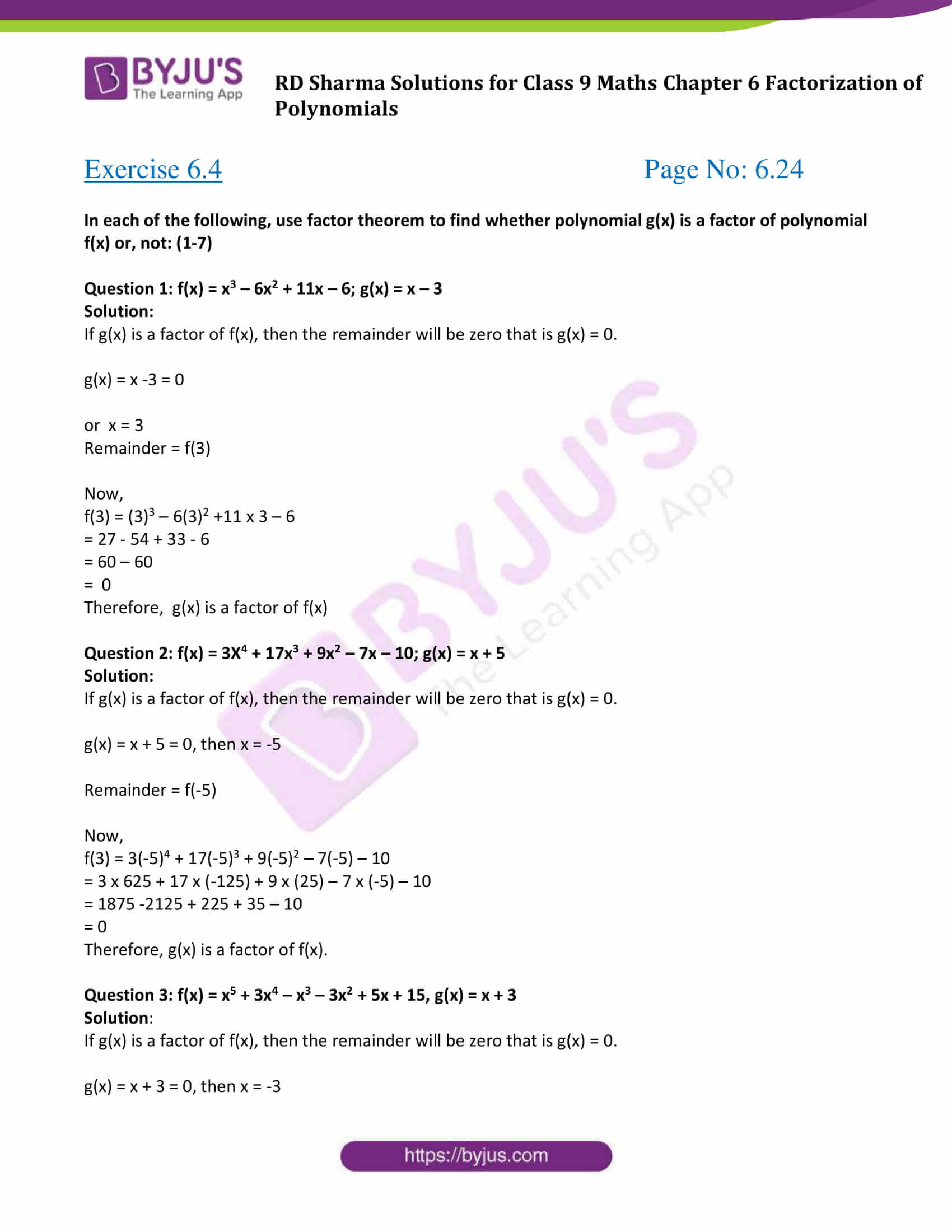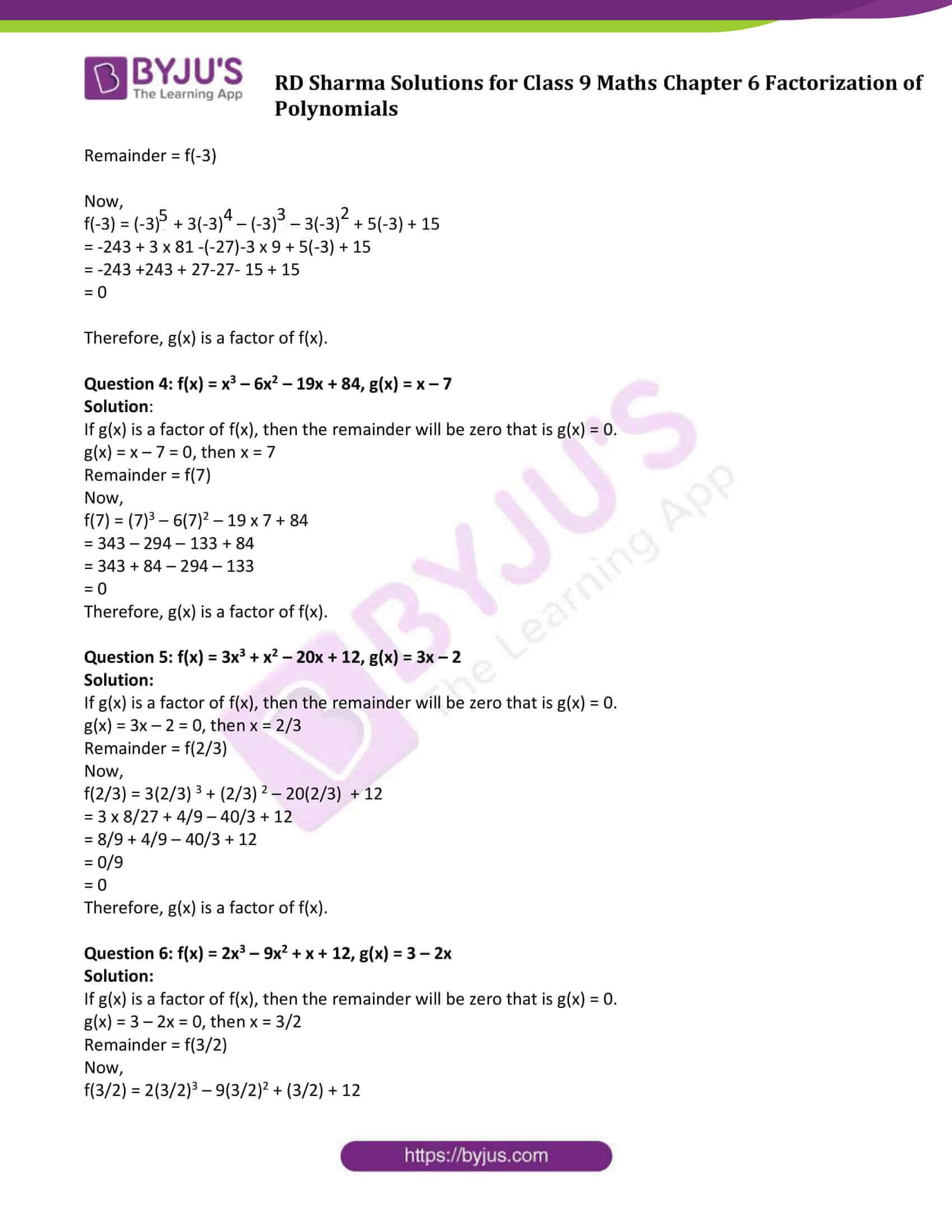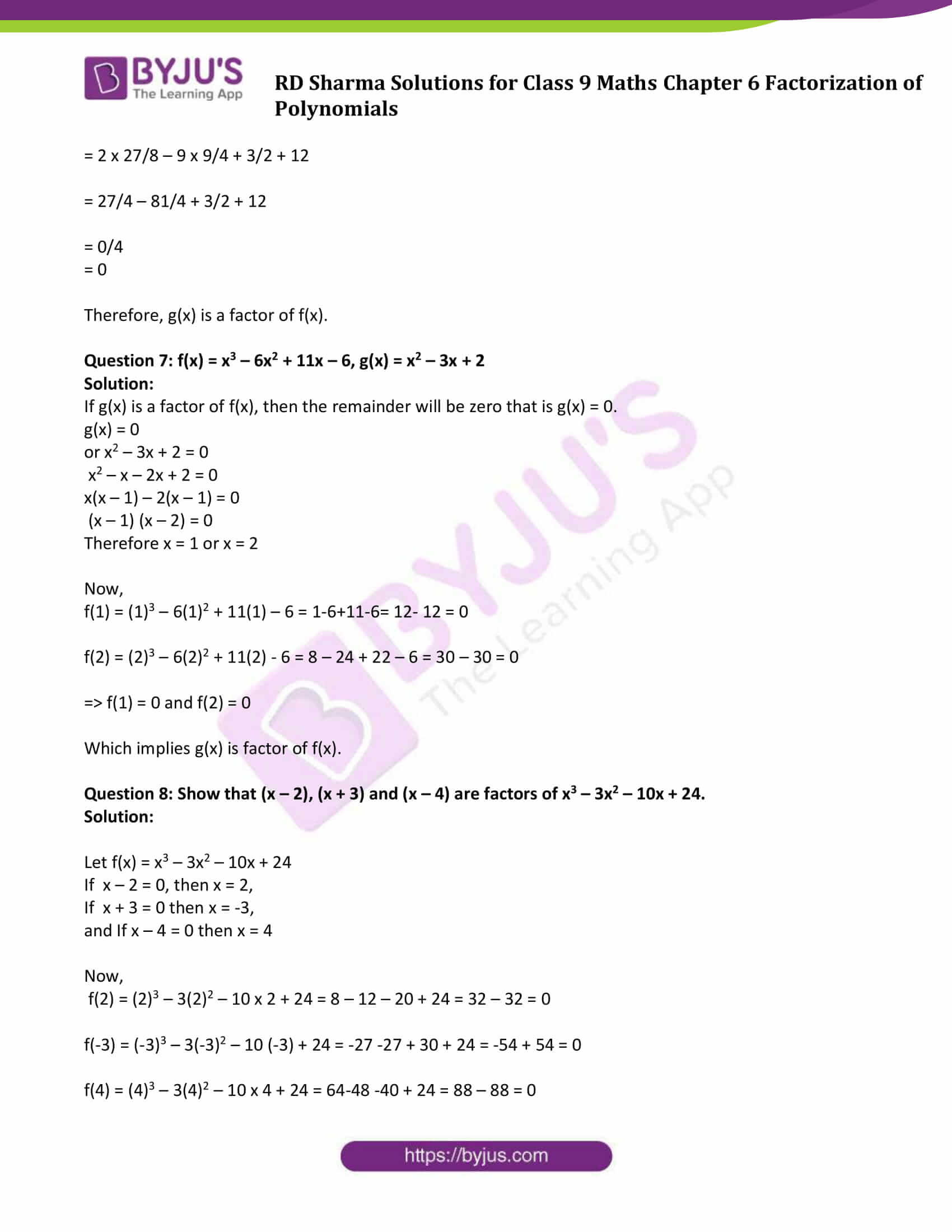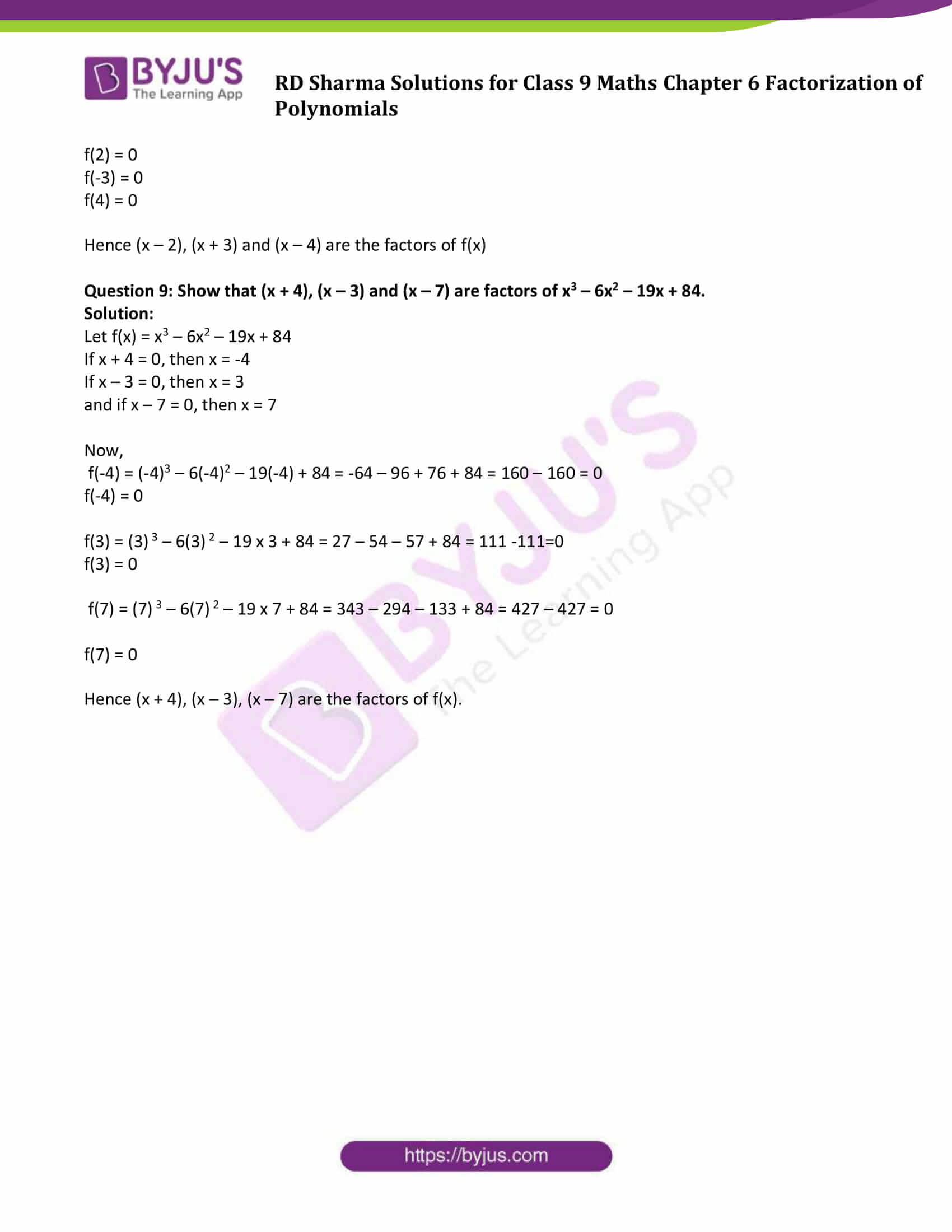# RD Sharma Solutions for Class 9 Maths Chapter 6 Factorization of Polynomials Exercise 6.4

RD Sharma Class 9 Mathematics Chapter 6 Exercise 6.4 Factorization of Polynomials is provided here. This exercise dealt with factor theorem. According to the theorem, let f(x) be a polynomial of degree greater than or equal to one and a be a real number such that f(a) = 0, then (x – a) is a factor of f(x). Conversely, if (x – a) is a factor of f(x), then f(a) = 0.

For more details, students can practice RD Sharma Solutions Class 9 Chapter 6 .

## Download PDF of RD Sharma Solutions for Class 9 Maths Chapter 6 Factorization of Polynomials Exercise 6.4### Access Answers to Maths RD Sharma Solutions for Class 9 Chapter 6 Factorization of Polynomials Exercise 6.4 Page number 6.24

#### Exercise 6.4 Page No: 6.24

In each of the following, use factor theorem to find whether polynomial g(x) is a factor of polynomial f(x) or, not: (1-7)

Question 1: f(x) = x3 – 6x2 + 11x – 6; g(x) = x – 3

Solution:

If g(x) is a factor of f(x), then the remainder will be zero that is g(x) = 0.

g(x) = x -3 = 0

or x = 3

Remainder = f(3)

Now,

f(3) = (3)3 – 6(3)2 +11 x 3 – 6

= 27 – 54 + 33 – 6

= 60 – 60

= 0

Therefore, g(x) is a factor of f(x)

Question 2: f(x) = 3X4 + 17x3 + 9x2 – 7x – 10; g(x) = x + 5

Solution:

If g(x) is a factor of f(x), then the remainder will be zero that is g(x) = 0.

g(x) = x + 5 = 0, then x = -5

Remainder = f(-5)

Now,

f(3) = 3(-5)4 + 17(-5)3 + 9(-5)2 – 7(-5) – 10

= 3 x 625 + 17 x (-125) + 9 x (25) – 7 x (-5) – 10

= 1875 -2125 + 225 + 35 – 10

= 0

Therefore, g(x) is a factor of f(x).

Question 3: f(x) = x5 + 3x4 – x3 – 3x2 + 5x + 15, g(x) = x + 3

Solution:

If g(x) is a factor of f(x), then the remainder will be zero that is g(x) = 0.

g(x) = x + 3 = 0, then x = -3

Remainder = f(-3)

Now,

f(-3) = (-3)5 + 3(-3)4 – (-3)3 – 3(-3)2 + 5(-3) + 15

= -243 + 3 x 81 -(-27)-3 x 9 + 5(-3) + 15

= -243 +243 + 27-27- 15 + 15

= 0

Therefore, g(x) is a factor of f(x).

Question 4: f(x) = x3 – 6x2 – 19x + 84, g(x) = x – 7

Solution:

If g(x) is a factor of f(x), then the remainder will be zero that is g(x) = 0.

g(x) = x – 7 = 0, then x = 7

Remainder = f(7)

Now,

f(7) = (7)3 – 6(7)2 – 19 x 7 + 84

= 343 – 294 – 133 + 84

= 343 + 84 – 294 – 133

= 0

Therefore, g(x) is a factor of f(x).

Question 5: f(x) = 3x3 + x2 – 20x + 12, g(x) = 3x – 2

Solution:

If g(x) is a factor of f(x), then the remainder will be zero that is g(x) = 0.

g(x) = 3x – 2 = 0, then x = 2/3

Remainder = f(2/3)

Now,

f(2/3) = 3(2/3) 3 + (2/3) 2 – 20(2/3) + 12

= 3 x 8/27 + 4/9 – 40/3 + 12

= 8/9 + 4/9 – 40/3 + 12

= 0/9

= 0

Therefore, g(x) is a factor of f(x).

Question 6: f(x) = 2x3 – 9x2 + x + 12, g(x) = 3 – 2x

Solution:

If g(x) is a factor of f(x), then the remainder will be zero that is g(x) = 0.

g(x) = 3 – 2x = 0, then x = 3/2

Remainder = f(3/2)

Now,

f(3/2) = 2(3/2)3 – 9(3/2)2 + (3/2) + 12

= 2 x 27/8 – 9 x 9/4 + 3/2 + 12

= 27/4 – 81/4 + 3/2 + 12

= 0/4

= 0

Therefore, g(x) is a factor of f(x).

Question 7: f(x) = x3 – 6x2 + 11x – 6, g(x) = x2 – 3x + 2

Solution:

If g(x) is a factor of f(x), then the remainder will be zero that is g(x) = 0.

g(x) = 0

or x2 – 3x + 2 = 0

x2 – x – 2x + 2 = 0

x(x – 1) – 2(x – 1) = 0

(x – 1) (x – 2) = 0

Therefore x = 1 or x = 2

Now,

‍f(1) = (1)3 – 6(1)2 + 11(1) – 6 = 1-6+11-6= 12- 12 = 0

f(2) = (2)3 – 6(2)2 + 11(2) – 6 = 8 – 24 + 22 – 6 = 30 – 30 = 0

=> f(1) = 0 and f(2) = 0

‍Which implies g(x) is factor of f(x).

Question 8: Show that (x – 2), (x + 3) and (x – 4) are factors of x3 – 3x2 – 10x + 24.

Solution:

Let f(x) = x3 – 3x2 – 10x + 24

If x – 2 = 0, then x = 2,

If x + 3 = 0 then x = -3,

and If x – 4 = 0 then x = 4

Now,

f(2) = (2)3 – 3(2)2 – 10 x 2 + 24 = 8 – 12 – 20 + 24 = 32 – 32 = 0

f(-3) = (-3)3 – 3(-3)2 – 10 (-3) + 24 = -27 -27 + 30 + 24 = -54 + 54 = 0

f(4) = (4)3 – 3(4)2 – 10 x 4 + 24 = 64-48 -40 + 24 = 88 – 88 = 0

f(2) = 0

f(-3) = 0

f(4) = 0

Hence (x – 2), (x + 3) and (x – 4) are the factors of f(x)

Question 9: Show that (x + 4), (x – 3) and (x – 7) are factors of x3 – 6x2 – 19x + 84.

Solution:

Let f(x) = x3 – 6x2 – 19x + 84

If x + 4 = 0, then x = -4

If x – 3 = 0, then x = 3

and if x – 7 = 0, then x = 7

Now,

f(-4) = (-4)3 – 6(-4)2 – 19(-4) + 84 = -64 – 96 + 76 + 84 = 160 – 160 = 0

f(-4) = 0

f(3) = (3) 3 – 6(3) 2 – 19 x 3 + 84 = 27 – 54 – 57 + 84 = 111 -111=0

f(3) = 0

f(7) = (7) 3 – 6(7) 2 – 19 x 7 + 84 = 343 – 294 – 133 + 84 = 427 – 427 = 0

f(7) = 0

Hence (x + 4), (x – 3), (x – 7) are the factors of f(x).

## RD Sharma Solutions for Class 9 Maths Chapter 6 Factorization of Polynomials Exercise 6.4

RD Sharma Solutions Class 9 Maths Chapter 6 Factorization of Polynomials Exercise 6.4 is based on Factor Theorem. This exercise solution is prepared by subject experts using simple and step by step problem-solving approach to clear students doubts on the concept.